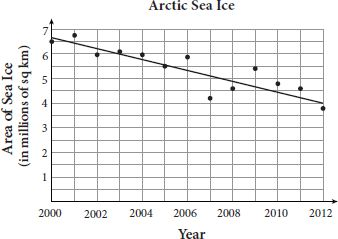# SAT Math Multiple Choice Question 852: Answer and Explanation

### Test Information

Question: 852

12. Sea ice extent is a measurement of the area of ocean with at least 15% sea ice. Thegraph below shows data for the extent of Arctic sea ice between 2000 and 2012 as reportedby the National Snow and Ice Data Center. The line of best fit is also shown. Theequation of the line is A(t) = -0.187t + 380.5, where A(t) represents the area of measurable sea ice in the Arctic Ocean in the year t.Based on the equation of the line of best fit, which of the following statements accuratelydescribes the change in the amount of measurable Arctic sea ice during the given timeperiod?

• A. The amount of sea ice increased approximately 187,000 square kilometers per year.
• B. The amount of sea ice increased approximately 187 million square kilometers per year.
• C. The amount of sea ice decreased approximately 187,000 square kilometers per year.
• D. The amount of sea ice decreased approximately 187 million square kilometers per year.